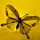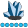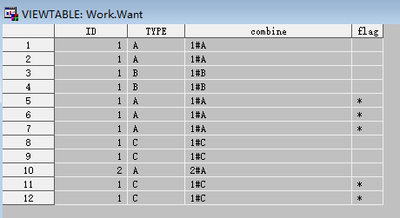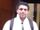## How to count until there is a change in a variable

I have some data that looks like the below:

ID  TYPE

1     A

1     A

1     B

1     B

1     A     *

1     A     *

1     A     *

1     C

1     C

2     A

...

This data is already sorted by  a 3rd variable TIME, which is not shown here. Basically I was to flag when the count of a particular combination of ID and TYPE is greater than 2 (see starred rows). It is possible for example that 1A is followed by 1B but the count of each is less than or equal to two, I wouldnt flag those. I only want to flag it if they occur more than twice consecutively in the sequence it is already sorted by.

8 REPLIES 8

## Re: How to count until there is a change in a variable

There are probably smarter ways to do this but here is one possibility:

``````data Have;
input ID \$ Type \$;
datalines;
1     A
1     A
1     B
1     B
1     A
1     A
1     A
1     C
1     C
2     A
;
run;

data Have2;
set Have;
by ID;
if first.ID then Type_Count = 1;
retain Last_Type;
else do;
if Type = Last_Type then Type_Count + 1;
else Type_Count = 1;
if Type_Count >= 3 then Type_Count_Flag = 'Y';
end;
Last_Type = Type;
if Type_Count = 1 then Group_ID + 1;
Order + 1;
run;

proc sql;
create table Want as
select A.ID
,A.Type
,B.Type_Count_Flag
from Have2 as A
left join Have2 as B
on A.Group_ID = B.Group_ID
and B.Type_Count_Flag = 'Y'
order by A.Order;
quit;``````whymath
Lapis Lazuli | Level 10

## Re: How to count until there is a change in a variable

You can use a lookup array:

``````data have;
input ID TYPE\$;
cards;
1 A
1 A
1 B
1 B
1 A
1 A
1 A
1 C
1 C
2 A
1 C
1 C
;
run;

%let nobs=&sysnobs;
data want;
set have;
by id type notsorted;

combine=catx('#',id,type);
array _par_[&nobs.]\$_temporary_;
retain flag;

if first.type then do;
if combine in _par_ then flag='*';
else do;
_par_[_n_]=combine;
flag='';
end;
end;
run;
``````If ID and TYPE combination have been appeared in the array, flag it, if not, push this combination into array. And be caution to do this at first.TYPE, or it is more difficult to consider the consecutive ID and TYPE combinations.whymath
Lapis Lazuli | Level 10

## Re: How to count until there is a change in a variable

Shift! I got it wrong. Here is the right anwser:

``````data want;
do until(last.type);
set have;
by id type notsorted;
sum=sum(sum,1);
end;

do until(last.type);
set have;
by id type notsorted;
if sum>2 then flag='*';
output;
end;
run;``````

## Re: How to count until there is a change in a variable

hm its not working, its flagging everything for me because its sorting by ID and TYPE regardless of the TIME variable.whymath
Lapis Lazuli | Level 10

## Re: How to count until there is a change in a variable

Could you please post an example data with the TIME variable? And write down what is your desired output in this example, too.

## Re: How to count until there is a change in a variable

@SAS_Muggle wrote:
hm its not working, its flagging everything for me because its sorting by ID and TYPE regardless of the TIME variable.

Why did it SORT by ID and TYPE?

Did you accidentally add an unwanted PROC SORT step?
Is the data coming from some external database that is doing the sorting on the fly?

## Re: How to count until there is a change in a variable

I assume that your data is sorted by ID and TIME, and if you do not sort the data by ID and TYPE before the data step, the solution proposed by @whymath should work (note the NOTSORTED option on the BY statement) - or this one, which makes the count in the loop header:

``````data want;
do _N_=1 by 1 until(last.type);
set have;
by id type notsorted;
end;
if _N_>2 then flag ='*';
do until(last.type);
set have;
by id type notsorted;
output;
end;
run;``````novinosrin
Tourmaline | Level 20

## Re: How to count until there is a change in a variable

Hi @SAS_Muggle , Assuming my understanding of your requirement is correct,  methinks your question presents a neat use case for Hash suminc method. Of course, Hash requires significant RAM, nevertheless should be quick.

``````
data Have;
input ID \$ Type \$;
datalines;
1     A
1     A
1     B
1     B
1     A
1     A
1     A
1     C
1     C
2     A
;
run;

data want ;
retain _iorc_ 1 ;
if _n_=1 then do;
dcl hash h (suminc:'_iorc_') ;
h.definekey('id','type' ) ;
h.definedone( );
end ;
set have ;
h.ref ( ) ;
h.sum (sum : _count) ;
if _count > 2 then flag = '*' ;
drop _: ;
run ;``````
Discussion stats
• 8 replies
• 557 views
• 5 likes
• 6 in conversation